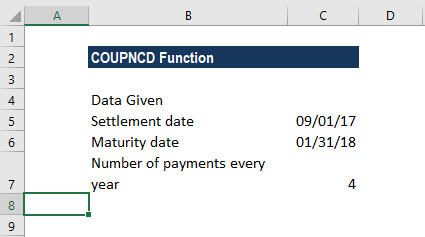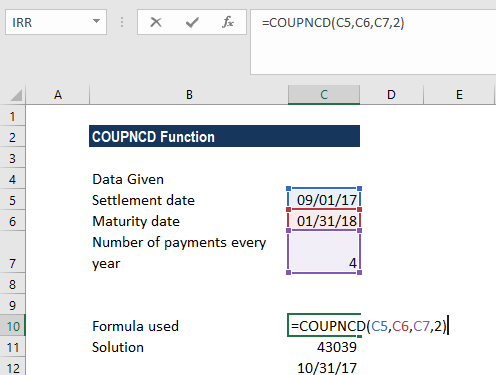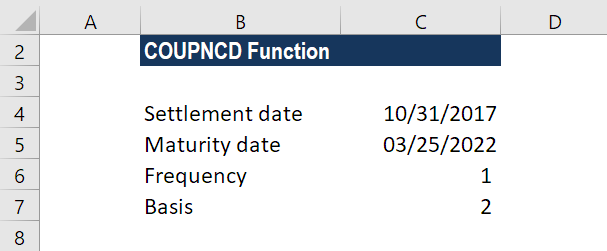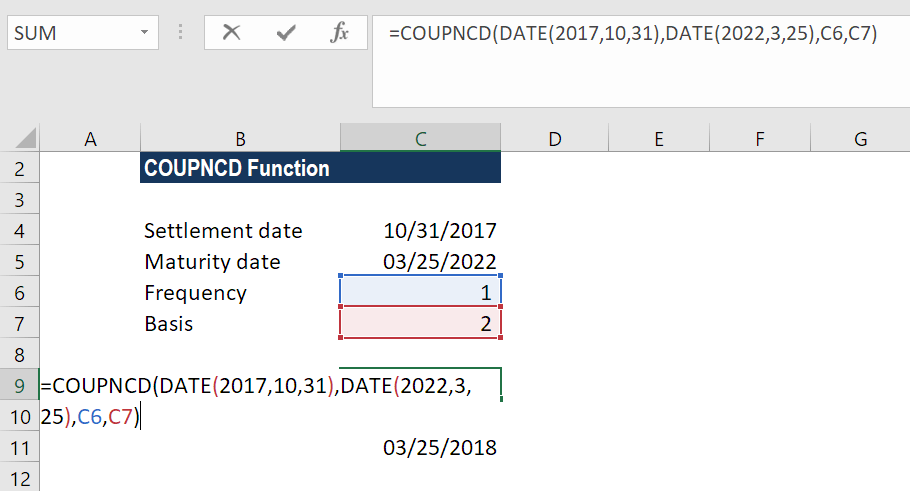# COUPNCD Function

Calculates the next coupon date after settlement

## What is the COUPNCD Function?

The COUPNCD Function is an Excel financial function. It calculates the number that is the next coupon date after settlement. After purchasing a bond, we can calculate the date when the first coupon payment is due using COUPNCD.

### Formula

=COUPNCD(settlement, maturity, frequency, [basis])

The COUPNCD function uses the following arguments:

1. Settlement (required argument) – This is the settlement date of a given security. It is the date after the security is traded to the buyer.
2. Maturity (required argument) – This is the date when the security expires.
3. Frequency (required argument) – The number of coupon payments per year. The argument can take a value of 1 (annual payment), 2 (semi-annual payments), or 4 (quarterly payments).
4. Basis (optional argument) – This specifies the day count basis to be used. It uses one of the following values:

BasisDay Count basis
0 or omittedUS(NASD) 30/360
1Actual/actual
2Actual/360
3Actual/365
4European 30/360

The function will default to zero when omitted. It indicates that the days in the month are counted using the US 30-day method with a 360-day year. When we enter 1 as the basis, the function uses the actual number of days in the month and year. Whereas, when we enter 2, it will count the actual days in the month with a 360-day year, while 3 will assume a 365-day year. When we enter 4 as the basis, it is the same as 1 except that it uses the European 30-day method.

### How to use the COUPDNCD Function in Excel?

To understand the uses of the COUPNCD function, let’s consider a few examples:

#### Example 1

Let’s see how the function works when we are given the following data:Additionally, we are told that the count basis should be 2.

Using the formula =COUPNCD(C5,C6,C7,2), we get 43039 as the result.As we are aware, Excel store dates in number format. So, we can use the format cell and convert it into date format as shown in cell C12.

#### Example 2

As the COUPNCD function doesn’t accept dates in text format, we need to convert them into a DATE format. Let’s see an example to understand it. Suppose we are given the following data:As we need to find the number of days using the function, we need to first convert the dates given in text format.

The formula to be used would be =COUPDAYS(DATE(2017,10,31),DATE(2022,3,25),1,2).The result we get here is 43184, which is March 25, 2018. Excel first converted the dates in text format into proper dates and then calculated the number of days. Here, we used 1 as the frequency and 2 as the basis.

### Few notes about the COUPNCD function

1. #NUM! error – Occurs in the following scenarios:
• When the settlement date provided is greater than or equal to (≥) the maturity date.
• When the given frequency argument provided by the user is not equal to 1, 2, or 4.
• When the given basis argument is a number other than 0, 1, 2, 3 or 4.
2. #VALUE! error – Occurs in the following scenarios:
• When the given settlement date or the maturity date is not a valid date. Remember that we need to enter dates in date format or else use the DATE function to convert them into proper dates. The function doesn’t work when dates given are in text format. For example, =COUPNCD(“1/25/2023″,”11/15/2024”,2,1) = #VALUE.
• Any of the arguments given is non-numeric.
3. The COUPNCD function truncates all arguments to integers.

Thanks for reading CFI’s guide to the COUPNCD Excel function. By taking the time to learn and master Excel functions, you’ll significantly speed up your financial analysis. To learn more, check out these additional CFI resources:

• Excel Functions for Finance